Next: 3D Motion Model - Up: Representation Previous: 3D Structure Model

### 3D Motion Model - Translation

The translational motion is represented as the 3-D location of the object reference frame relative to the current camera reference frame using the vector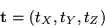The tX and tY components correspond to directions parallel to the image plane, while the tZ component corresponds to the depth of the object along the optical axis. As such, the sensitivity of image plane motion to tX and tY motion will be similar to each other, while the sensitivity to tZ motion will differ, to a level dependent upon the focal length of the imaging geometry.

For typical video camera focal lengths, even with wide angle'' lenses, there is already much less sensitivity to tZ motion than there is to (tX, tY) motion. For longer focal lengths the sensitivity decreases until in the limiting orthographic case there is zero image plane sensitivity to tZ motion.

For this reason, tZ cannot be represented explicitly in our estimation process. Instead, the product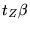is estimated. The coordinate frame transformation equation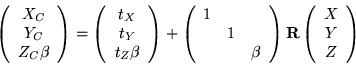(15)

combined with Equation 12 demonstrates that onlyis actually required to generate an equation for the image plane measurements (u,v) as a function of the motion, structure, and camera parameters (rotation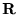is discussed below).

Furthermore, the sensitivity ofdoes not degenerate at long focal lengths as does tZ. For example, the sensitivities of the u image coordinate to both tZ andare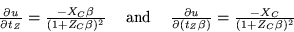demonstrating thatremains observable from the measurements and is therefore estimable for long focal lengths, while tZ is not (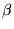approaches zero for long focal lengths).

Thus we parameterize translation with the vector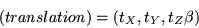True translation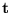can be recovered post-estimation simply by dividing out the focal parameter from. This is valid only ifis non-zero (non-orthographic), which is desirable, because tZ is not geometrically recoverable in the orthographic case. To see this mathematically, the error variance on tZ will be the error variance onscaled by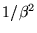, which gets large for narrow fields of view.Next: 3D Motion Model - Up: Representation Previous: 3D Structure Model

1999-05-17